# Roman Numerals

In this mini-lesson, we will explore the world of Roman numerals. We will do so by finding answers to what is Roman numerals chart, how to identify Roman numerals symbols, how to read Roman numerals, what is X or M in Roman numerals, and how to perform Roman numeral conversion while discovering the interesting facts around them.

Kabir has an extremely old, grandfather clock in his living room, but he finds it difficult to read the time. Do you know why? It's because, instead of natural numbers, the clock has Roman numerals in it. For reading the clock, Kabir has to learn about Roman numerals.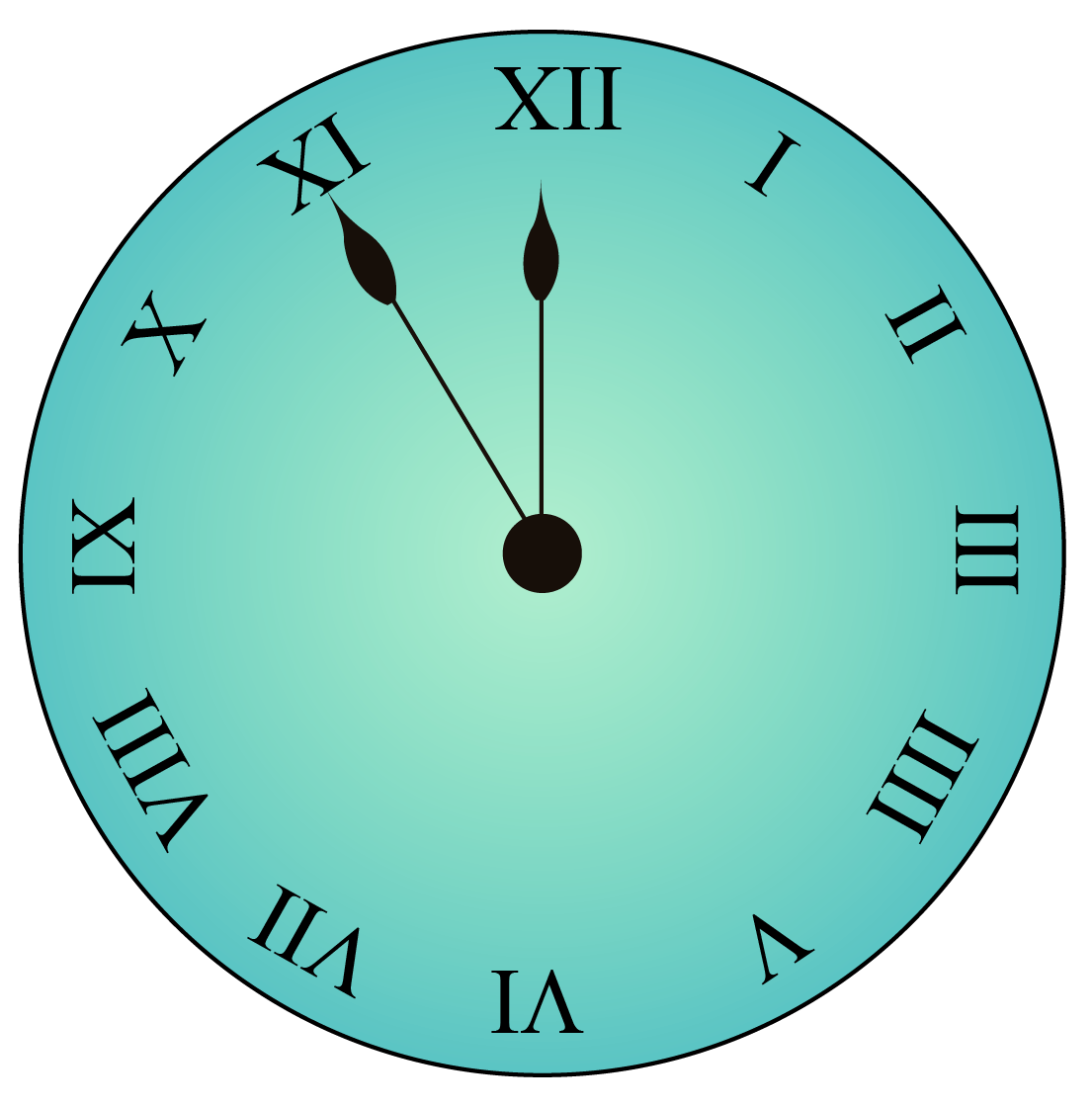Let us all learn with Kabir about Roman Numerals.

## Lesson Plan

 1 What Are Roman Numerals? 2 Solved Examples on Roman Numerals 3 Interactive Questions on Roman Numerals

## What Are Roman Numerals?

Roman numerals are one of its kind number system, which originated centuries ago in ancient Rome.

Roman numerals symbols were commonly used in denoting numbers and remained widely popular late into the Middle Ages.

If you want to learn how to read Roman numerals, all you need to know are alphabets.

The Roman numerals list comprises letters taken from the Latin alphabets, which as we know it, starts from A and ends at Z.

It’s not hard to spot Roman numerals around. You may find them at the bottom of text pages too.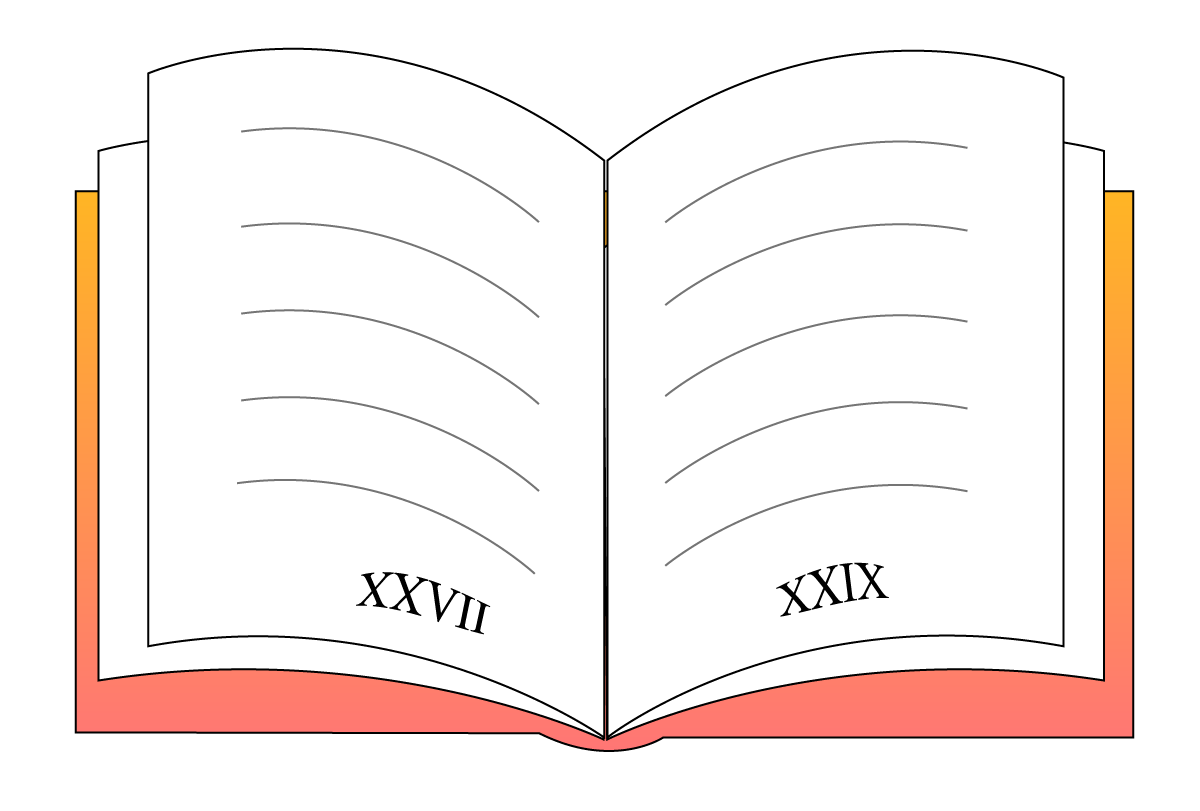## Fundamentals of Roman Numerals

If you know these 7 basic Roman numeral conversions, you can easily convert all numbers to Roman numerals.

Decimal Number

Roman Numeral Symbol

1

$$I$$

5

$$V$$

10

$$X$$

50

$$L$$

100

$$C$$

500

$$D$$

1000

$$M$$

In addition to these basic conversions, there are a couple of rules you need to be watchful of while converting digits to Roman numerals and vice versa.

Let’s understand those rules with examples.

## Solved Examples

 Example 1

Krish wants to know if it's time for his favorite show. The shorthand of the clock points at $$VIII$$. What hour is it?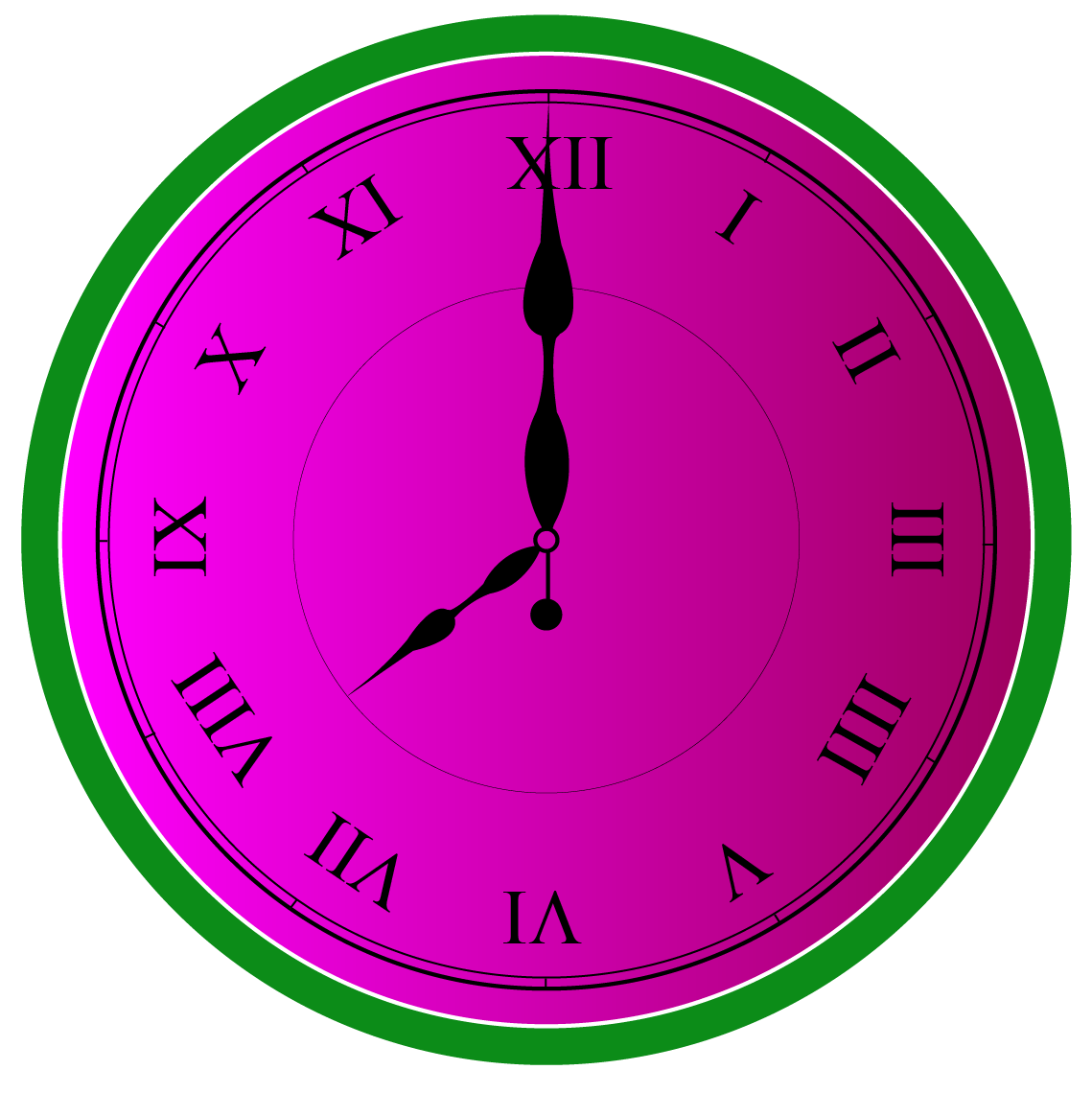Solution

We know that $$V$$ stands for 5 and I stands for 1

Since I is the smaller symbol and appears thrice after $$V$$, it will get added to $$V$$.

$VIII=V+I+I+I$

Thus, the time is 8 o'clock.

 $$\therefore$$ $$VIII=5+1+1+1=8$$
 Example 2

The long hand of Anushka's clock is pointed at $$IX$$. She wants to know the minute count.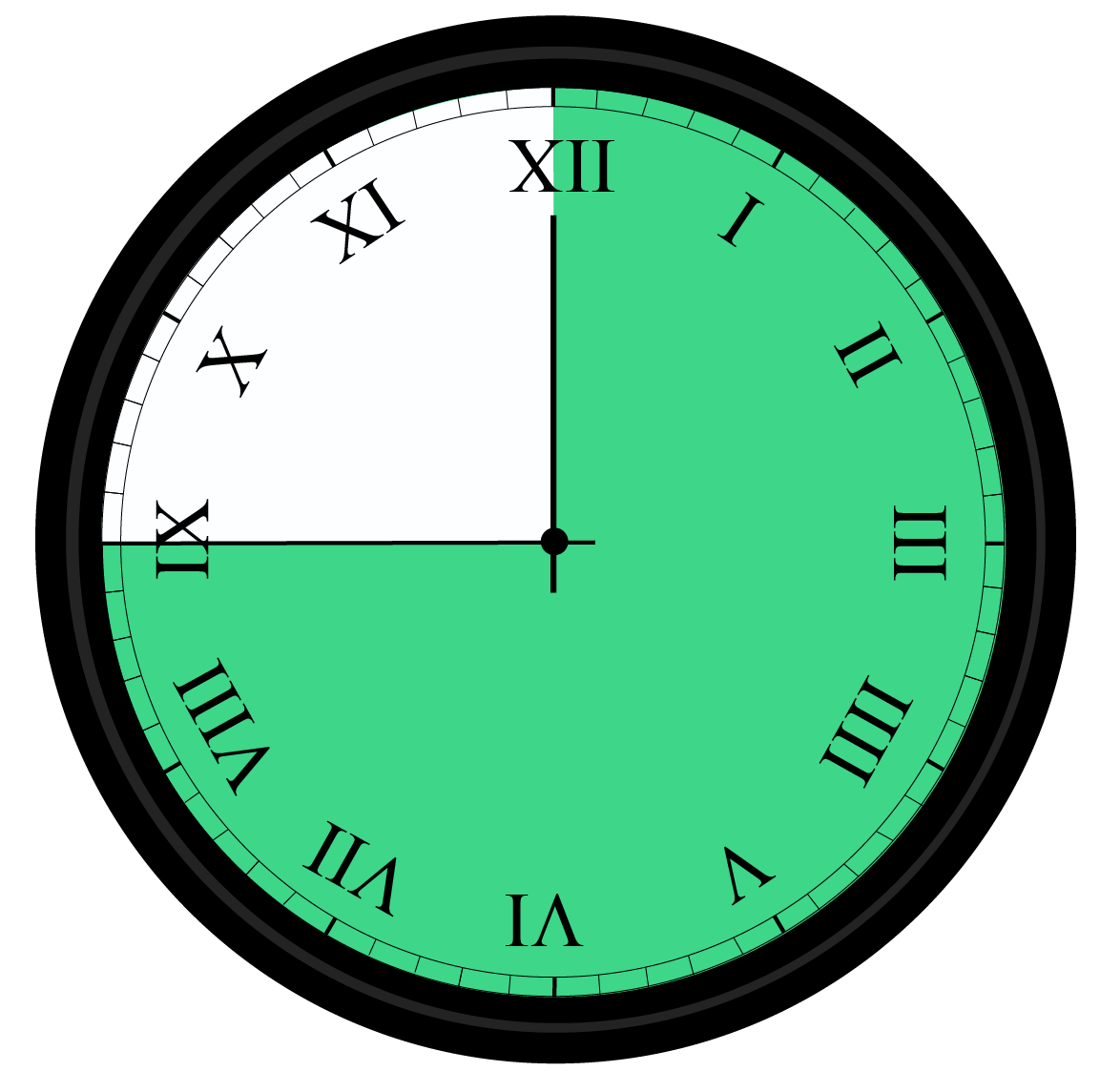Solution

If the smaller symbol appears before the larger symbol, it will get subtracted from the smaller symbol.

$$IX$$ becomes $$X-I$$, as X in Roman numerals is 10

Thus, the minute count is

 $$\therefore$$ $$IX =10-1= 9$$

 Example 3

Ekta was asked to represent a Roman numeral using both addition and subtraction. If the Roman numeral is $$XIX$$, how will she represent it in a decimal number system?

Solution

Going from right to left, the last two symbols are $$IX$$, which is  $$- 1 + 10$$ or $$9$$

$$X$$ in Roman numerals is $$10$$

 $$\therefore$$ $$XIX=10 -1 + 10 = 19$$
 Example 4

The teacher asks Ritvik to open page number 28, but the page numbers are in Roman numerals. How will Ritvik identify the right page?Solution

The number 28 can be broken down to $$10 + 10 + 5 + 1 +1 + 1$$

It is done keeping in mind that the biggest Roman symbol 28 can have is 10, which is $$X$$.

The next biggest Roman numeral will be 5, and the last will be 1.

 $$\therefore$$ $$28=XXVIII$$
 Example 5

How would you write the year 2019 in Roman numerals?

Solution

The biggest symbol in 2019 is 1000, which is $$M$$.

We will break down the number further.

2019 becomes $$1000 + 1000 + 10 – 1 + 10$$

 $$\therefore$$ $$2019 =MMXIX$$Tips and Tricks
1. Don’t use the Roman symbol more than thrice in a number. If there is a requirement for more, use subtraction by placing $$I$$ before the bigger symbol.
2. Identify the biggest symbol, in the beginning, to solve the problem easily.
3. While converting from Roman numerals to decimal numbers, look out for $$IV$$, which is 4, and $$IX$$, which is 9. Identifying them right away will make your task simpler.
4. Roman numerals are only applicable for natural numbers, and not fractions, decimals, or other real numbers.

## Roman Numerals Chart

A Roman numerals chart can be useful in doing conversions for all Roman numbers.

This is what it looks like.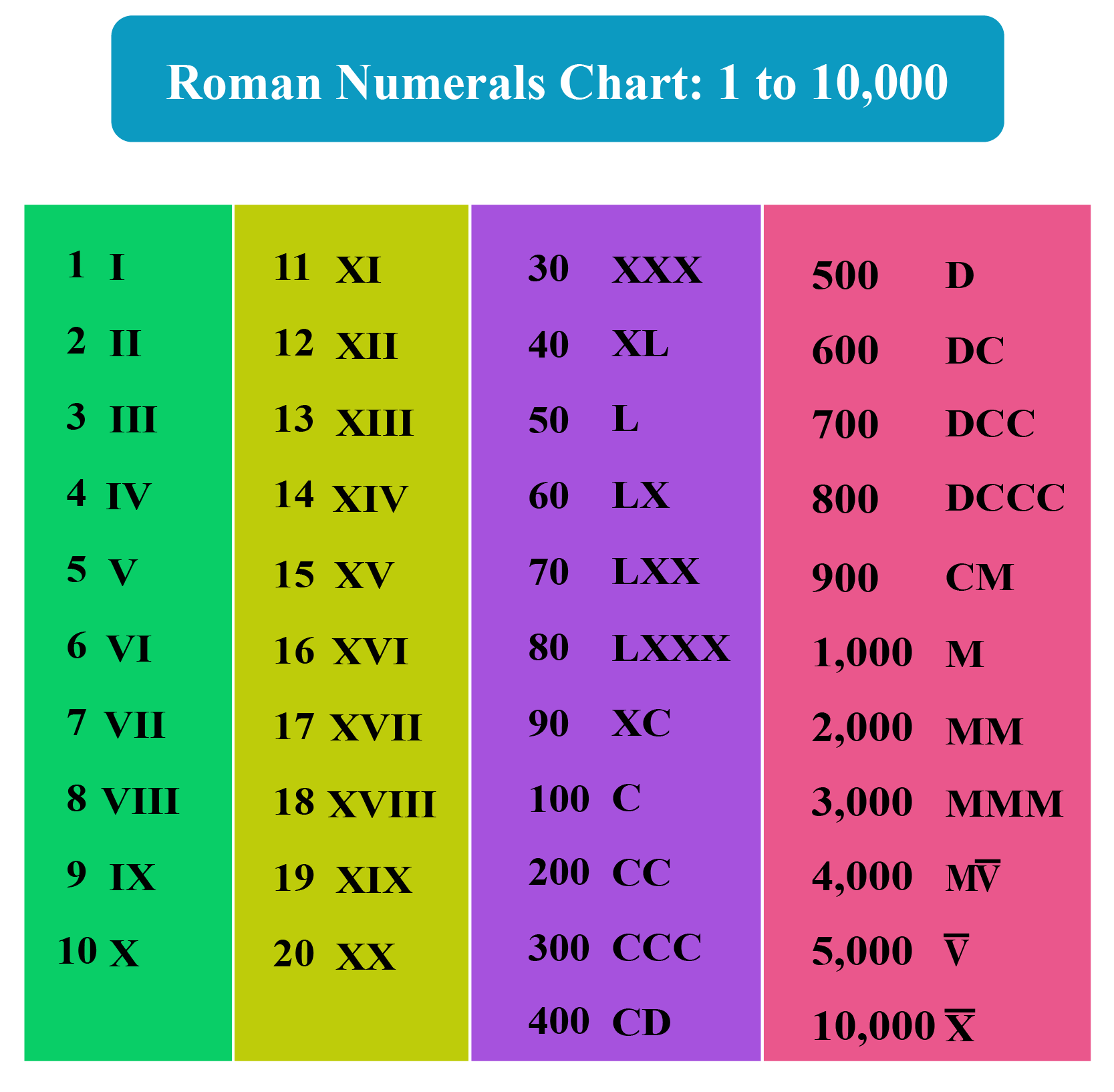More Important Topics
Numbers
Algebra
Geometry
Measurement
Money
Data
Trigonometry
Calculus